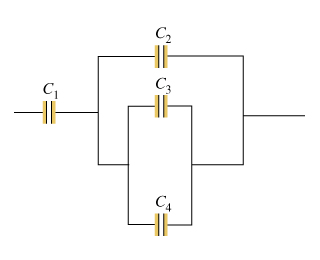# Problem: Consider the combination of capacitors shown in the diagram, where C1 = 3.00 μF , C2 = 11.0 μF , C3 = 3.00 μF , and C4 = 5.00 μF . (Figure 1)Find the equivalent capacitance CA of the network of capacitors. Express your answer in microfarads.

🤓 Based on our data, we think this question is relevant for Professor Khatri's class at University of the District of Columbia.

###### FREE Expert Solution

Equivalent capacitance for capacitors in parallel:

$\overline{){{\mathbit{C}}}_{\mathbf{e}\mathbf{q}}{\mathbf{=}}{{\mathbit{C}}}_{{\mathbf{1}}}{\mathbf{+}}{{\mathbit{C}}}_{{\mathbf{2}}}{\mathbf{+}}{{\mathbit{C}}}_{{\mathbf{3}}}}$

Equivalent capacitance for capacitors in series:

$\overline{)\frac{\mathbf{1}}{{\mathbf{C}}_{{\mathbf{eq}}}}{\mathbf{=}}\frac{\mathbf{1}}{{\mathbf{C}}_{{\mathbf{1}}}}{\mathbf{+}}\frac{\mathbf{1}}{{\mathbf{C}}_{{\mathbf{2}}}}{\mathbf{+}}\frac{\mathbf{1}}{{\mathbf{C}}_{{\mathbf{3}}}}}$

C3 and C4 are in parallel:

C3,4 = (C3 + C4) = (3.00 + 5.00) = 8.00  μF###### Problem Details

Consider the combination of capacitors shown in the diagram, where C1 = 3.00 μF , C2 = 11.0 μF , C3 = 3.00 μF , and C4 = 5.00 μF . (Figure 1)